Publicité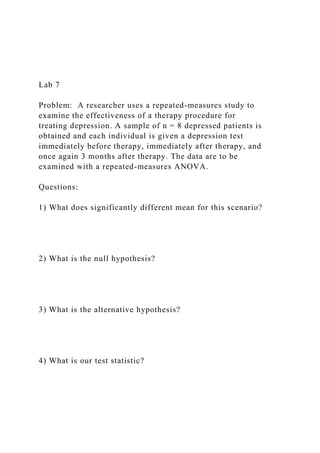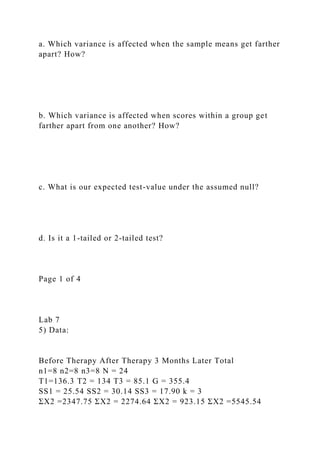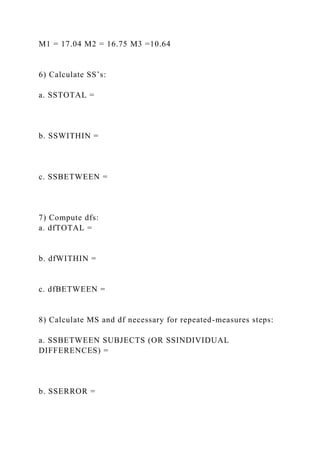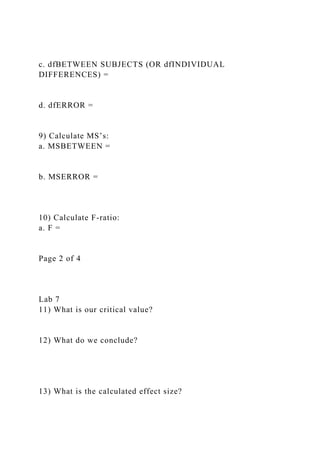Publicité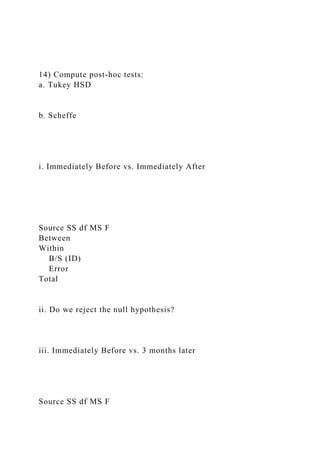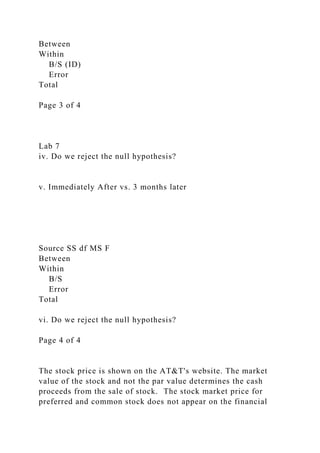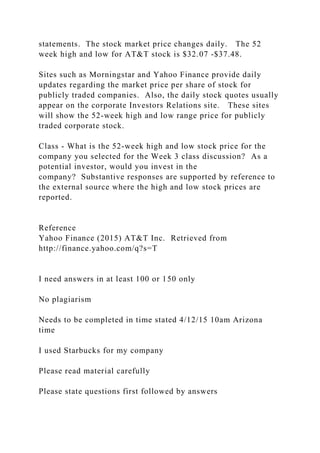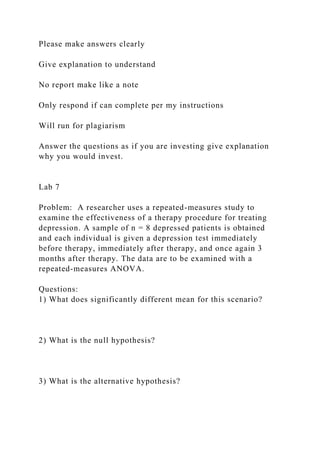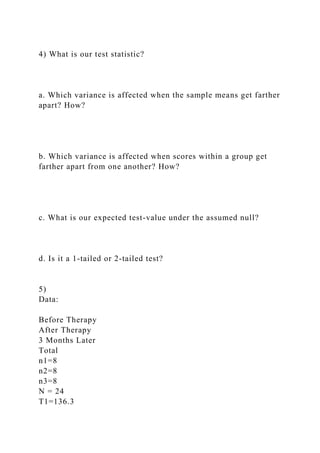Publicité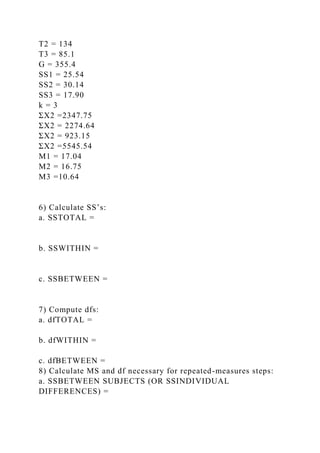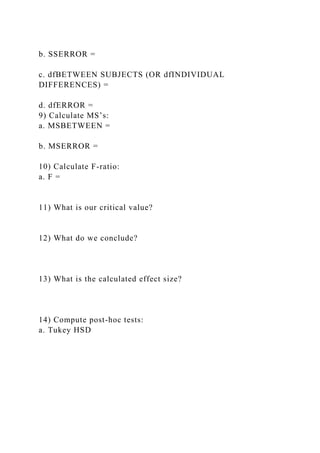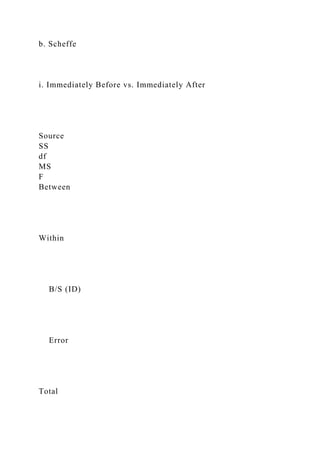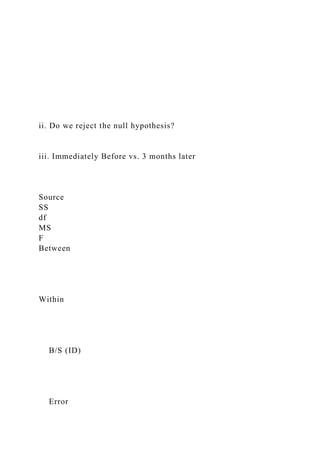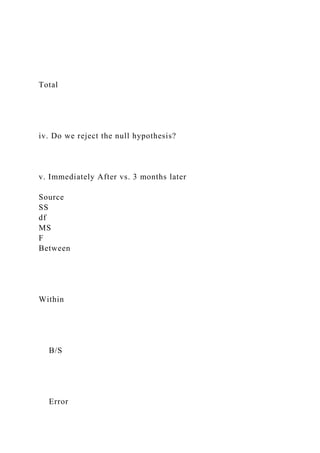Publicité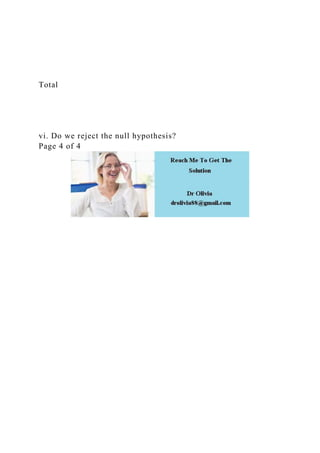Prochain SlideShare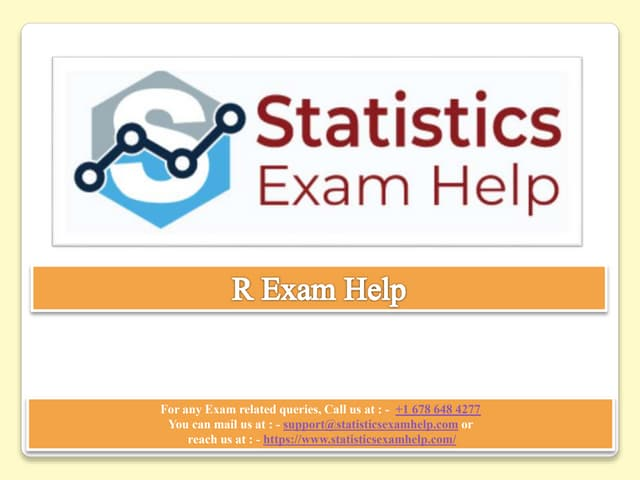R Exam Help
Chargement dans ... 3
1 sur 15
Publicité

### Lab 7 Problem A researcher uses a repeated-measures stu.docx

1. Lab 7 Problem: A researcher uses a repeated-measures study to examine the effectiveness of a therapy procedure for treating depression. A sample of n = 8 depressed patients is obtained and each individual is given a depression test immediately before therapy, immediately after therapy, and once again 3 months after therapy. The data are to be examined with a repeated-measures ANOVA. Questions: 1) What does significantly different mean for this scenario? 2) What is the null hypothesis? 3) What is the alternative hypothesis? 4) What is our test statistic?
2. a. Which variance is affected when the sample means get farther apart? How? b. Which variance is affected when scores within a group get farther apart from one another? How? c. What is our expected test-value under the assumed null? d. Is it a 1-tailed or 2-tailed test? Page 1 of 4 Lab 7 5) Data: Before Therapy After Therapy 3 Months Later Total n1=8 n2=8 n3=8 N = 24 T1=136.3 T2 = 134 T3 = 85.1 G = 355.4 SS1 = 25.54 SS2 = 30.14 SS3 = 17.90 k = 3 ΣX2 =2347.75 ΣX2 = 2274.64 ΣX2 = 923.15 ΣX2 =5545.54
3. M1 = 17.04 M2 = 16.75 M3 =10.64 6) Calculate SS’s: a. SSTOTAL = b. SSWITHIN = c. SSBETWEEN = 7) Compute dfs: a. dfTOTAL = b. dfWITHIN = c. dfBETWEEN = 8) Calculate MS and df necessary for repeated-measures steps: a. SSBETWEEN SUBJECTS (OR SSINDIVIDUAL DIFFERENCES) = b. SSERROR =
4. c. dfBETWEEN SUBJECTS (OR dfINDIVIDUAL DIFFERENCES) = d. dfERROR = 9) Calculate MS’s: a. MSBETWEEN = b. MSERROR = 10) Calculate F-ratio: a. F = Page 2 of 4 Lab 7 11) What is our critical value? 12) What do we conclude? 13) What is the calculated effect size?
5. 14) Compute post-hoc tests: a. Tukey HSD b. Scheffe i. Immediately Before vs. Immediately After Source SS df MS F Between Within B/S (ID) Error Total ii. Do we reject the null hypothesis? iii. Immediately Before vs. 3 months later Source SS df MS F
6. Between Within B/S (ID) Error Total Page 3 of 4 Lab 7 iv. Do we reject the null hypothesis? v. Immediately After vs. 3 months later Source SS df MS F Between Within B/S Error Total vi. Do we reject the null hypothesis? Page 4 of 4 The stock price is shown on the AT&T's website. The market value of the stock and not the par value determines the cash proceeds from the sale of stock. The stock market price for preferred and common stock does not appear on the financial
7. statements. The stock market price changes daily. The 52 week high and low for AT&T stock is \$32.07 -\$37.48. Sites such as Morningstar and Yahoo Finance provide daily updates regarding the market price per share of stock for publicly traded companies. Also, the daily stock quotes usually appear on the corporate Investors Relations site. These sites will show the 52-week high and low range price for publicly traded corporate stock. Class - What is the 52-week high and low stock price for the company you selected for the Week 3 class discussion? As a potential investor, would you invest in the company? Substantive responses are supported by reference to the external source where the high and low stock prices are reported. Reference Yahoo Finance (2015) AT&T Inc. Retrieved from http://finance.yahoo.com/q?s=T I need answers in at least 100 or 150 only No plagiarism Needs to be completed in time stated 4/12/15 10am Arizona time I used Starbucks for my company Please read material carefully Please state questions first followed by answers
8. Please make answers clearly Give explanation to understand No report make like a note Only respond if can complete per my instructions Will run for plagiarism Answer the questions as if you are investing give explanation why you would invest. Lab 7 Problem: A researcher uses a repeated-measures study to examine the effectiveness of a therapy procedure for treating depression. A sample of n = 8 depressed patients is obtained and each individual is given a depression test immediately before therapy, immediately after therapy, and once again 3 months after therapy. The data are to be examined with a repeated-measures ANOVA. Questions: 1) What does significantly different mean for this scenario? 2) What is the null hypothesis? 3) What is the alternative hypothesis?
9. 4) What is our test statistic? a. Which variance is affected when the sample means get farther apart? How? b. Which variance is affected when scores within a group get farther apart from one another? How? c. What is our expected test-value under the assumed null? d. Is it a 1-tailed or 2-tailed test? 5) Data: Before Therapy After Therapy 3 Months Later Total n1=8 n2=8 n3=8 N = 24 T1=136.3
10. T2 = 134 T3 = 85.1 G = 355.4 SS1 = 25.54 SS2 = 30.14 SS3 = 17.90 k = 3 ΣX2 =2347.75 ΣX2 = 2274.64 ΣX2 = 923.15 ΣX2 =5545.54 M1 = 17.04 M2 = 16.75 M3 =10.64 6) Calculate SS’s: a. SSTOTAL = b. SSWITHIN = c. SSBETWEEN = 7) Compute dfs: a. dfTOTAL = b. dfWITHIN = c. dfBETWEEN = 8) Calculate MS and df necessary for repeated-measures steps: a. SSBETWEEN SUBJECTS (OR SSINDIVIDUAL DIFFERENCES) =
11. b. SSERROR = c. dfBETWEEN SUBJECTS (OR dfINDIVIDUAL DIFFERENCES) = d. dfERROR = 9) Calculate MS’s: a. MSBETWEEN = b. MSERROR = 10) Calculate F-ratio: a. F = 11) What is our critical value? 12) What do we conclude? 13) What is the calculated effect size? 14) Compute post-hoc tests: a. Tukey HSD
12. b. Scheffe i. Immediately Before vs. Immediately After Source SS df MS F Between Within B/S (ID) Error Total
13. ii. Do we reject the null hypothesis? iii. Immediately Before vs. 3 months later Source SS df MS F Between Within B/S (ID) Error
14. Total iv. Do we reject the null hypothesis? v. Immediately After vs. 3 months later Source SS df MS F Between Within B/S Error
15. Total vi. Do we reject the null hypothesis? Page 4 of 4
Publicité